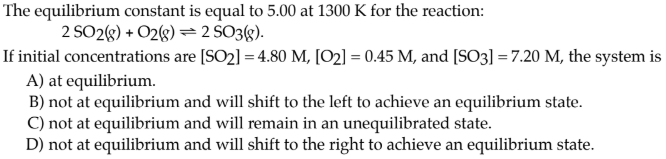# The equilibrium constant is equal to 5.00 at 1300 K for the reaction: 2 SO2(g) + O2(g) ⇌ 2 SO3(g). If initial concentrations are [SO2] = 4.80 M, [O2] = 0.45 M, and [SO3] = 7.20 M, the system is A) at equilibrium. B) not at equilibrium and will shift to the left to achieve an equilibrium state. C) not at equilibrium and will remain in an unequilibrated state. D) not at equilibrium and will shift to the right to achieve an equilibrium state.